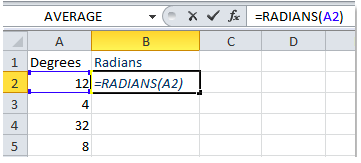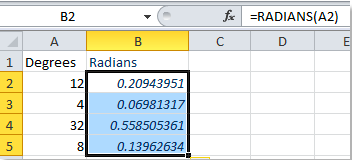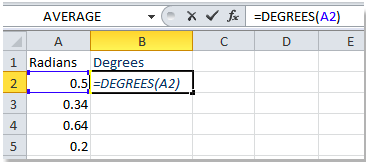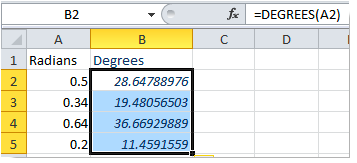Get instant live expert help with Excel or Google Sheets“My Excelchat expert helped me in less than 20 minutes, saving me what would have been 5 hours of work!”

#### Post your problem and you’ll get expert help in seconds.

Your message must be at least 40 characters
Our professional experts are available now. Your privacy is guaranteed.

# How to convert radians to degrees in Excel – Excelchat

When working with Excel to solve trigonometric equations, we are required to measure the angles in radians rather than degrees. But not everyone knows how to change Excel from radians to degrees. This might pose a challenge when one is working to solve lots of trigonometric questions. In this post, will look at the easy ways through which one can convert Excel degrees to radians.

To convert radians to degrees, we can simply take advantage of the Excel RADIANS function which converts degrees to radians. Also, we can use the Excel DEGREES function if we want to convert radians to degrees as well.

Converting degrees to radians with the Excel RADIANS function should be the easiest thing anyone can do. All you need to have is a list of your data in an Excel spreadsheet, have a column of your data that you want to convert into radians and then another one where you want the radians to appear. Have a look at figure 1 below;Figure 1: Converting degrees to radians

As you can see from the above figure, we need to have the Degrees column as well as the radians column. The next thing is to select a cell in the radians column where we want to have our result in radians. The cell should be next to that of the degrees that we want to convert.

Then type in the Radians formula as below;

`=RADIANS (A2)`

After typing in the formula, hit enter.Figure 2: Degrees and radians

The last thing we need to do in order to get all the radians of the degrees in our workbook is to copy down the formula across all the other cells.

## Converting radians to degrees with the DEGREES function

We can also convert radians to degrees using the DEGREES function in Excel. This works almost the same way as the RADIANS function. Look at the example below to see how we can easily have Excel convert radians to degrees.Figure 3: Converting radians to degrees

And when we press enter and copy down the formula, we shall populate all the other cells with degrees as shown in figure 4 below;Figure 4: Radians to degrees

## Instant Connection to an Expert through our Excelchat Service

Most of the time, the problem you will need to solve will be more complex than a simple application of a formula or function. If you want to save hours of research and frustration, try our live Excelchat service! Our Excel Experts are available 24/7 to answer any Excel question you may have. We guarantee a connection within 30 seconds and a customized solution within 20 minutes.